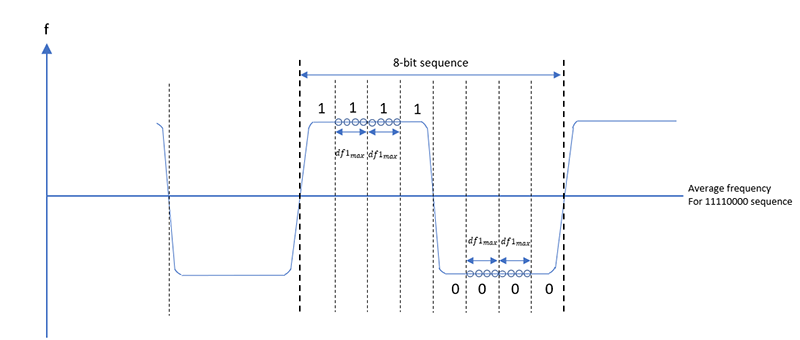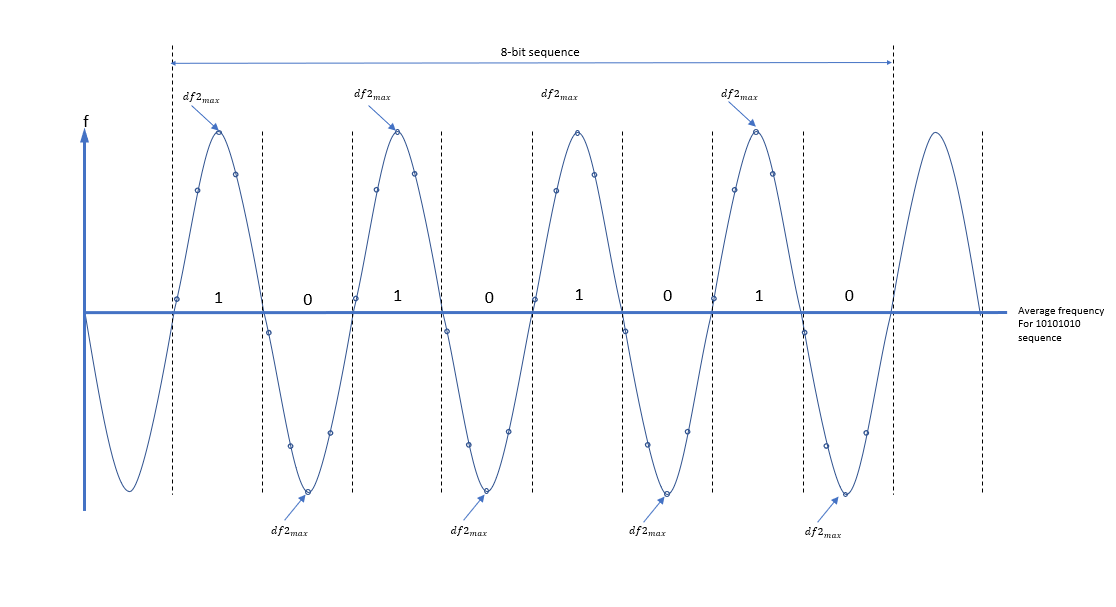From 09:00 PM Friday, August 7 - 10:30 AM CST Saturday, August 8, ni.com will be undergoing system upgrades that may result in temporary service interruption.

We appreciate your patience as we improve our online experience.

# df1 and df2

RFmx Bluetooth 19.1 Help

Edition Date: October 2019

Part Number: 377889C-01

»View Product InfoRFmx Bluetooth 19.1 HelpRFmx Bluetooth 20.0 Help

In Bluetooth standards, Basic Rate (BR) and Low Energy (LE), the packets use Gaussian Frequency Shift Keying (GFSK) modulation scheme. Modulation accuracy of the Bluetooth transmitters that generate BR and LE packets are validated by verifying the frequency deviation values.

To realize the frequency measurement, it is required that the device under test (DUT) is set to generate the BR or LE packet with the maximum supported payload length and have 8-bit payload pattern 10101010 or 11110000 depending on the measurements type. The frequency deviation corresponding to the 11110000 pattern is called df1 measurement and the frequency deviation corresponding to the 10101010 pattern is called df2 measurement

The Bluetooth Special Interest Group (SIG) specifies the required values of the frequency deviation for the two payload patterns, 10101010 and 11110000. The frequency deviation limits also depend on whether the packet belongs to LE standard or BR standard. For the frequency deviation limits on BR packet, refer to section 4.5.7 of the Bluetooth Test specification RF.TS.5.1.0. For the frequency deviation limits on LE packet, refer to sections 4.4.3, 4.4.7 and 4.4.10 of the Bluetooth Test Specification RF-PHY.TS.5.1.1.

### RFmx Relevant Results

The relevant results provided by the RFmx measurements are as follows.

• ModAcc Results df1avg Mean (Hz)
• ModAcc Results df1avg Max (Hz)
• ModAcc Results df1avg Min (Hz)
• ModAcc Results Pk df1max Max (Hz)
• ModAcc Results Min df1max Min (Hz)
• ModAcc Results df2avg Mean (Hz)
• ModAcc Results df2avg Max (Hz)
• ModAcc Results df2avg Min (Hz)
• ModAcc Results Pk df2max Max (Hz)
• ModAcc Results Min df2max Min (Hz)
• ModAcc Results Percentage of Symbols above df2max Threshold (%)

To perform df1 measurement the DUT transmits BR or LE packet with full payload and 11110000 bit pattern. The tester acquires the signal and determines the zeroth bit position (p0) for each received packet to establish the timing reference for bits in the payload. Once the p0 position of the received packet has been established, the tester calculates the average frequency for each 00001111 8-bit sequence in the payload. The frequency deviation for each bit is determined by taking an average value across these samples. For each second, third, sixth and seventh of the 8 bits, the deviation from the average frequency within bit period is calculated and recorded as df1max. The average of all the df1max deviation values is calculated and recorded as df1avg.

## df1 Measurement

To perform df1 measurement the DUT transmits BR or LE packet with full payload and 11110000 bit pattern. The tester acquires the signal and determines the zeroth bit position (p0) for each received packet to establish the timing reference for bits in the payload. Once the p0 position of the received packet has been established, the tester calculates the average frequency for each â€œ00001111â€ 8-bit sequence in the payload. The frequency deviation for each bit is determined by taking an average value across these samples. For each second, third, sixth and seventh of the 8 bits, the deviation from the average frequency within bit period is calculated and recorded as df1max. The average of all the df1max deviation values is calculated and recorded as df1avg.Figure 1 - Frequency deviation measurement principle for 11110000 payload sequence

## df2 Measurement

A similar measurement procedure is performed while the DUT transmits a 10101010 bit pattern. However, in this case, for each bit maximum deviation from the average frequency is found and recorded as df2max. The average of all the df2max deviation values is calculated and recorded as df2avg. As a result, the maximum frequency deviation df2max and the average frequency deviation df2avg are determined for 01010101 bit pattern. Bluetooth specification requires the measurement to be performed over a period of at least 10 packets. In addition to the lowest operating frequency the modulation characteristic test should be conducted at mid operating frequency and the highest operating frequency of the DUT.Figure 2 - Frequency deviation measurement principle for 10101010 payload sequence